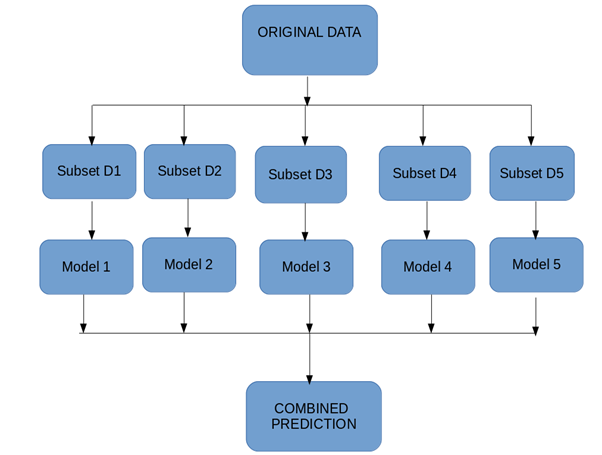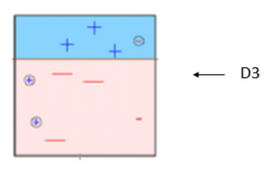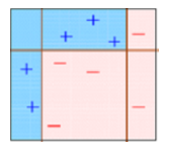# Bagging & Boosting – Theory

## Bagging

Bootstrap Aggregation (or Bagging for short), is a simple and very powerful ensemble method. Bootstrap method refers to random sampling with replacement. Here with replacement means a sample can be repetitive. Bagging allows model or algorithm to get understand about various biases and variance.

To create bagging model, first we create multiple random samples so that each new random sample will act as another (almost) independent dataset drawn from original distribution. Then, we can fit a weak learner for each of these samples and finally aggregate their outputs and obtain an ensemble model with less variance from its components.

Let’s understand it with an eg.as we can see in below figure where each sample population has different pieces and none of them are identical. This would then affect the overall mean, standard deviation and other descriptive metrics of a data set.  It develops more robust models.How bagging works

### How Bagging Works?

1. You generate multiple samples from your training set using next scheme: you take randomly an element from training set and then return it back. So, some of elements of training set will present multiple times in generated sample and some will be absent. These samples should have the same size as the train set.
2. You train you learner on each generated sample.
3. When you apply the algorithm you just average predictions of learners in case of regression or make the voting in case of classification.

Applying bagging often help to deal with overfitting by reducing prediction variance.

### Bagging Algorithms:

1. Take M bootstrap samples (with replacement)
2. Train M different classifiers on these bootstrap samples
3. For a new query, let all classifiers predict and take an average(or majority vote)
4. If the classifiers make independent errors, then their ensembles can improve performance.

## Boosting:

Boosting is an ensemble modeling technique which converts weak learner to strong learners.

Let’s understand it with an example. Let’s suppose you want to identify an email is a SPAM or NOT SPAM. To do that you need to take some criteria as follows.

1. Email has only one image file, It’s a SPAM
2. Email has only link, It’s a SPAM
3. Email body consist of sentence like “You won a prize money of \$ xxxx”, It’s a SPAM
4. Email from our official domain “datasciencelovers.com”, Not a SPAM
5. Email from known source, Not a SPAM

As we can see above there are multiple rules to identify an email is a spam or not. But if we will talk about individual rules they are not as powerful as multiple rules. There these individual rules is a weak learner.

To convert weak learner to strong learner, we’ll combine the prediction of each weak learner using methods like:
•   Using average/ weighted average
•   Considering prediction has higher vote

For example:  Above, we have defined 5 weak learners. Out of these 5, 3 are voted as ‘SPAM’ and 2 are voted as ‘Not a SPAM’. In this case, by default, we’ll consider an email as SPAM because we have higher (3) vote for ‘SPAM’

### Boosting Algorithm:

1. The base learner takes all the distributions and assigns equal weight or attention to each observation.
2. If there is any prediction error caused by first base learning algorithm, then we pay higher attention to observations having prediction error. Then, we apply the next base learning algorithm.
3. Iterate Step 2 till the limit of base learning algorithm is reached or higher accuracy is achieved.

Finally, it combines the outputs from weak learner and creates a strong learner which eventually improves the prediction power of the model.

### Types of Boosting Algorithm:

1. AdaBoost (Adaptive Boosting)
2. Gradient Tree Boosting
3. XGBoost

### AdaBoost(Adaptive Boosting)

Adaboost was the first successful and very popular boosting algorithm which developed for the purpose of binary classification. AdaBoost technique which combines multiple “weak classifiers” into a single “strong classifier”.

1. Initialise the dataset and assign equal weight to each of the data point.
2. Provide this as input to the model and identify the wrongly classified data points
3. Increase the weight of the wrongly classified data points.
4. if (got required results)
Go to step 5
else
Go to step 2
5. End

Let’s understand the concept with following example.

BOX – 1: In box 1 we have assigned equal weight to each data points and applied a decision stump to classify them as  + (plus) or – (minus). The decision stump (D1) has generated vertical line at left side to classify the data points. As we can see in the box vertical line has incorrectly predicted three + (plus) as – (minus). In this case, we will assign higher weights to these three + (plus) and apply another decision stump. As you can see in below image.Decision stump – 1

BOX – 2: Now in box 2 size of three incorrectly predicted + (plus) is bigger as compared to rest of the data points. In this case, the second decision stump (D2) will try to predict them correctly. Now, a vertical line (D2) at right side of this box has classified three mis-classified + (plus) correctly. But in this process, it has caused mis-classification errors again. This time with three -(minus). So we will assign higher weight to three – (minus) and apply another decision stump. As you can see in below image.Decision stump -2

BOX – 3: In box 3 there are three – (minus) has been given higher weights. A decision stump (D3) is applied to predict these mis-classified observation correctly. This time a horizontal line is generated to classify + (plus) and – (minus) based on higher weight of mis-classified observation.Decision stump – 3

BOX – 4: in box 4 we will combine D1, D2 and D3 to form a strong prediction having complex rule as compared to individual weak learner. As we can see this algorithm has classified these observation quite well as compared to any of individual weak learner.Decision Stump – 4

### Python Code

from sklearn.ensamble import AdaBoostClassifier
clf = AdaBoostClassifier(n_estimators=4, random_state=0, algorithm=’SAMME’)
clf.fit(X, Y)

• n_estimators : integer, optional (default=50)

The maximum number of estimators at which boosting is terminated. In case of perfect fit, the learning procedure is stopped early.

• random_state : int, RandomState instance or None, optional (default=None)
• algorithm : {‘SAMME’, ‘SAMME.R’}, optional (default=’SAMME.R’)

If ‘SAMME.R’ then use the SAMME.R real boosting algorithm. base estimator must support calculation of class probabilities. If ‘SAMME’ then use the SAMME discrete boosting algorithm.